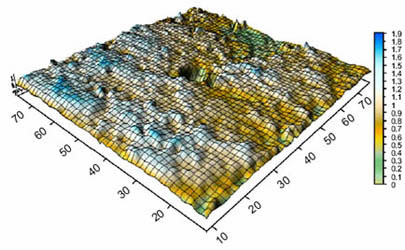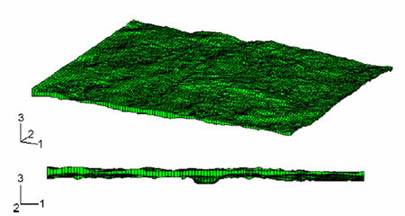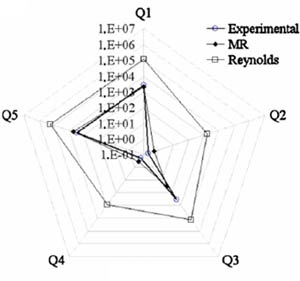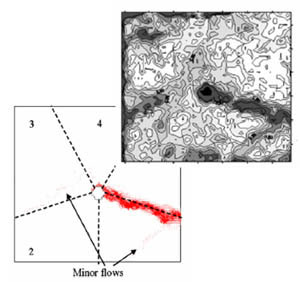Priority Research Centre for
GEOTECHNICAL SCIENCE AND ENGINEERING# Rock Joint Flow Behaviour

## Influence of Micro Roughness of a Rock Joint on the Macro Distribution of Flow

### Introduction

Understanding water flow through a rock mass is a fundamental issue in many areas of rock engineering. A rock mass is a dual permeability system where the flow through discontinuities is predominant. It is thus of prime importance to fully understand their hydromechanical behaviour to foresee how stress fields in situ can influence the fluid transport through fractures. Flow within a rock joint is governed by the Navier-Stokes equations which are very difficult to solve. A simplified version is the Reynolds equation, which is considered to give better flow prediction. A flow model based on the micro roughness of the rock joint has been proposed which gives a better prediction of flow anisotropy.

### Experimental Data

A comprehensive series of hydro-mechanical shear tests on rock joint after Hans with:

• Measurement of the rock morphology (Fig. 1)
• Measurement of inlet pressure and total flow rate.
• Measurement of the outlet flow rate (macro scale) in 5 sectors.

Capturing both rock wall morphologies at each step of shearing enables:

• Calculation of the roughness (JRC)
• Computation of the void space
• Calculation of mechanical aperture (Eel )

More details can be found in Hans J. & Boulon M. Int. J. Numer. Anal. Meth. Geomech 2003;27:513–48

### The Permeability Model

The void space, embedded between the rock walls and computed from both upper and lower morphologies, is described as a porous medium in which Darcy's law applies. (Fig.2)

A FEM calculation is performed using ABAQUS to compute the flow. The innovation here is the reduced coefficient of permeability given to each element, which incorporates a micro roughness effect.

The successive steps to calculate the reduced coefficient of permeability are summarized in the following:Figure 1: 3D representation of the morphological analysis of the lower rock wallFigure 2: 3D FEM representation of the void space embedded within both rock walls. 8715 elements.

### Results

Fig. 3 shows the distribution of water flowing out of a rock joint. The model developed (MR) fits the experimental results very well whereas the Reynolds equation gives an almost isotropic flow rate. Fig. 4 shows a good agreement between the measurement of void space obtained by the laser and the water distribution within the joint.Figure 3: Numerical and experimental distribution of flow rates per sectorFigure 4: Numerical distribution of water within the rock joint and experimential measurement of the void space

### Investigators

Research published in: Giacomini et al. Int. J. Rock Mech. & Min. Sci. Doi:10.1016/j.ijrmms.2007.04.007